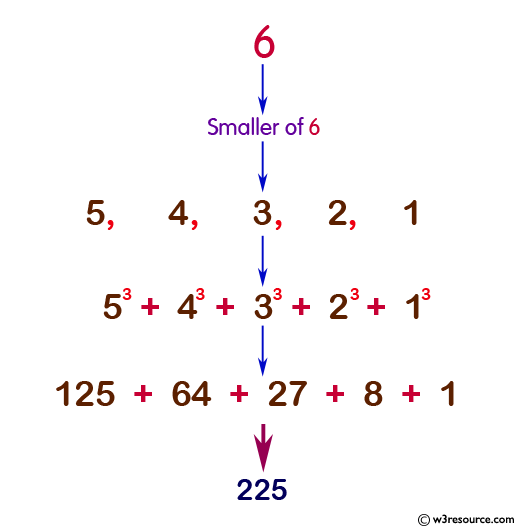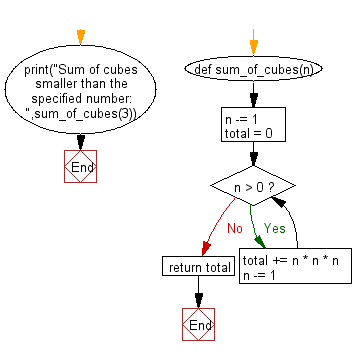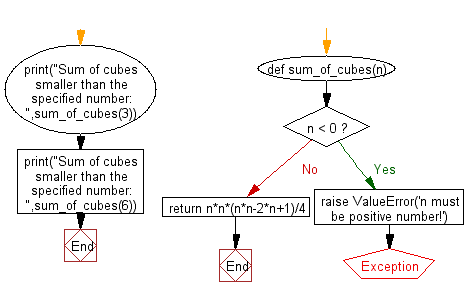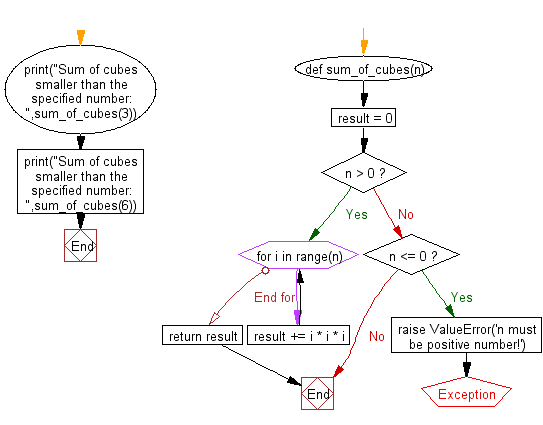﻿ Python: Function that takes a positive integer and returns the sum of the cube of all the positive integers smaller than the specified number - w3resource# Python: Function that takes a positive integer and returns the sum of the cube of all the positive integers smaller than the specified number

## Python Basic: Exercise-149 with Solution

Write a Python function that takes a positive integer and returns the sum of the cube of all the positive integers smaller than the specified number.

Ex.: 8 = 73+63+53+43+33+23+13 = 784

Sample Solution-1:

Python Code:

``````def sum_of_cubes(n):
n -= 1
total = 0
while n > 0:
total += n * n * n
n -= 1
print("Sum of cubes smaller than the specified number: ",sum_of_cubes(3))
``````

Sample Output:

```Sum of cubes smaller than the specified number:  9
```

Pictorial Presentation:Flowchart:## Visualize Python code execution:

The following tool visualize what the computer is doing step-by-step as it executes the said program:

Sample Solution-2:

Python Code:

``````def sum_of_cubes(n):
if n < 0:
raise ValueError('n must be positive number!')
return n*n*(n*n-2*n+1)/4
print("Sum of cubes smaller than the specified number: ",sum_of_cubes(3))
print("Sum of cubes smaller than the specified number: ",sum_of_cubes(6))
``````

Sample Output:

```Sum of cubes smaller than the specified number:  9.0
Sum of cubes smaller than the specified number:  225.0
```

Flowchart:## Visualize Python code execution:

The following tool visualize what the computer is doing step-by-step as it executes the said program:

Sample Solution-3:

Python Code:

``````def sum_of_cubes(n):
result = 0
if n > 0:
for i in range(n):
result += i * i * i
return result
elif n <= 0:
raise ValueError('n must be positive number!')
print("Sum of cubes smaller than the specified number: ",sum_of_cubes(3))
print("Sum of cubes smaller than the specified number: ",sum_of_cubes(6))
``````

Sample Output:

```Sum of cubes smaller than the specified number:  9
Sum of cubes smaller than the specified number:  225
```

Flowchart:## Visualize Python code execution:

The following tool visualize what the computer is doing step-by-step as it executes the said program:

Python Code Editor :

Have another way to solve this solution? Contribute your code (and comments) through Disqus.

What is the difficulty level of this exercise?

Test your Python skills with w3resource's quiz

﻿

## Python: Tips of the Day

Try-catch-else construct:

```try:
foo()
except Exception:
print("Exception occured")
else:
print("Exception didnt occur")
finally:
print("Always gets here")
```